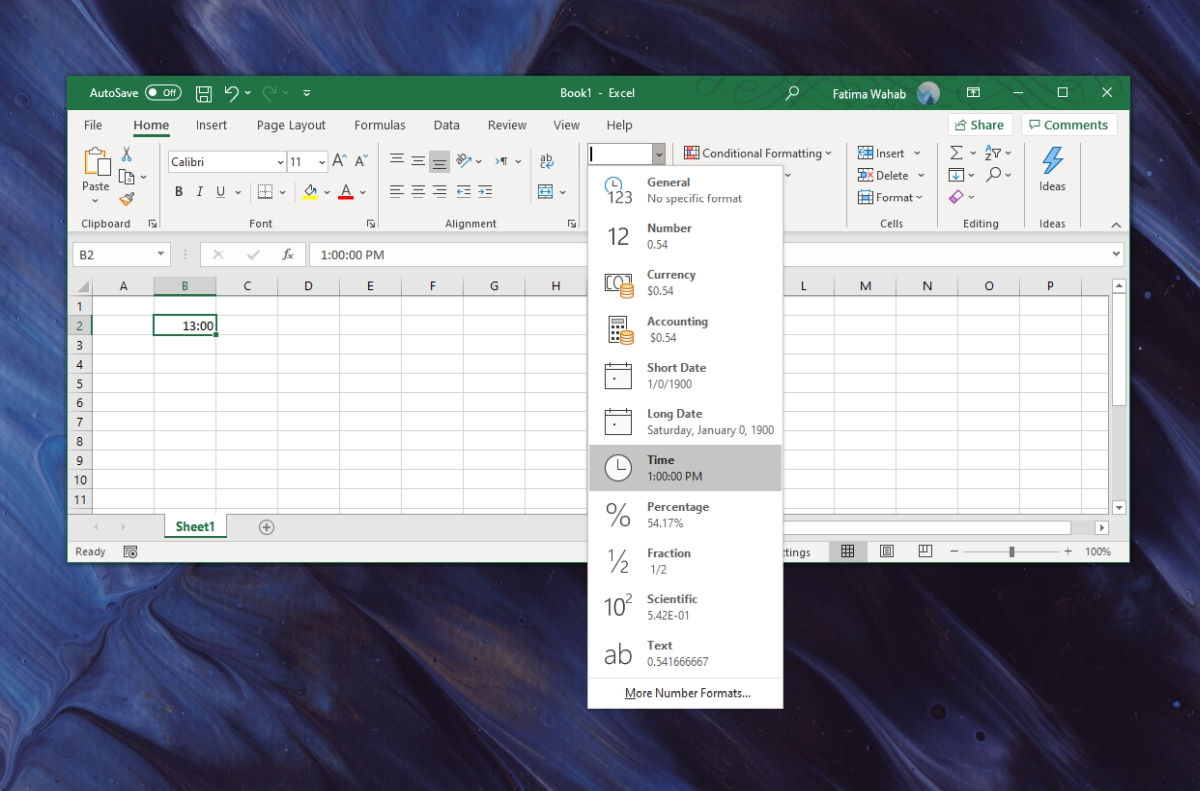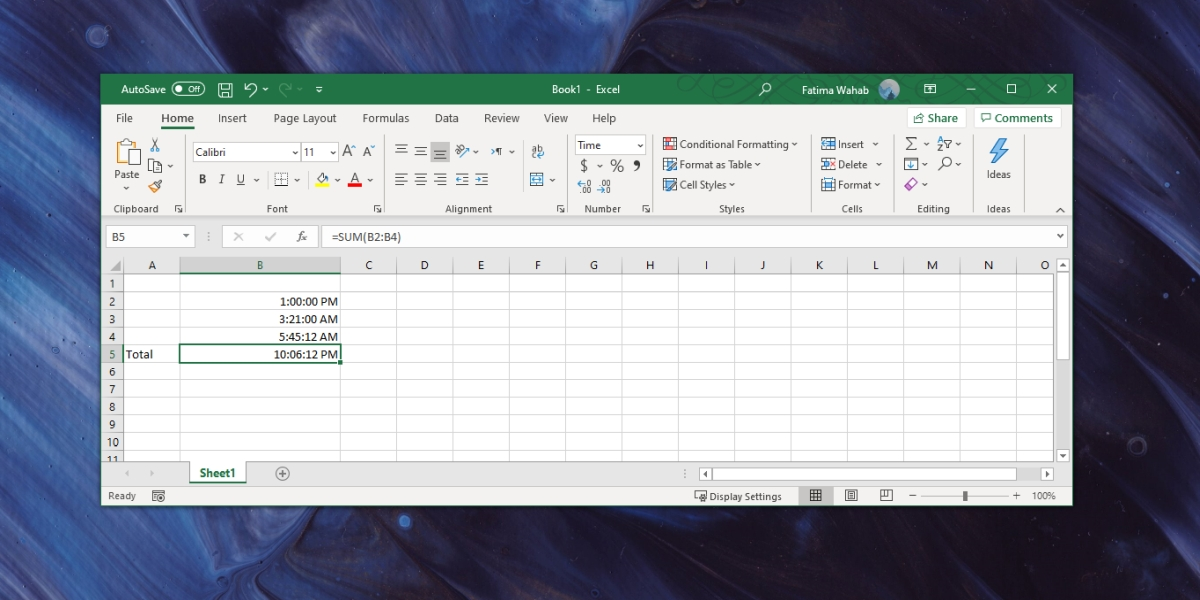# How to add hours in Microsoft Excel

Microsoft Excel can work with all types of data; numbers, percentages, fractions, currency, date, time, etc. You need to tell Excel what sort of data it is dealing with when you enter it in a cell but once you do, you can apply almost all simple and complex formulas to the data so long as Excel supports them and they can be applied to that particular type of data.

## Add hours in Microsoft Excel

We’re going to be covering two things here; how to record time in Excel i.e., how to tell the app that the value you’ve entered is not a number but a time value, and how to add multiple time entries.

### 1. Add time in Excel

In order to enter a time value in Excel, you need to do the following.

1. Open Excel.
2. Click inside a cell and enter time in the hh:mm:ss format e.g., 13:00:00 means 1 PM.
3. Select the cell (or an entire column if you plan on entering only time values in it).
4. On the Home tab, look for the Numbers toolbox.
5. Open the dropdown, and select Time.
6. The value in the cell (or column) will now be recognized as time.### 2. Sum/add hours in Excel

Let’s say you have a column full of various time-values and you need to add them together to see how much total time has been spent.

1. Make sure each cell has been set to be recognized as time.
2. Select an empty cell.
3. Go to the Home tab, and on the Numbers toolbox, open the dropdown.
4. Select Time.
5. Click inside the cell and enter the following formula to calculate total time. Num1 is the first cell that you want to add, Num2 is the second cell, and so on. Excel’s helpful autofill for formulas will show up and it’s easier to use.
`=sum(num1,num2,...)`
1. Tap Enter and you will get a total of the hours in the selected cells.## Conclusion

Microsoft Excel is one of the most commonly used spreadsheet apps. You will find it being used for all sorts of things e.g., inventory management, timekeeping, financial analysis, expensing, and more. For individual use, and for small-ish companies, it can serve multiple purposes and that’s precisely why it’s been built to work with so many different types of number data. The Sum isn’t the only formula you can apply to time values. You can also use the minus formula and find out difference in time, or you can multiply time by a number to find an estimate of hours/minutes that need to be spent on a task.

This site uses Akismet to reduce spam. Learn how your comment data is processed.